# Mathematics Commons™

20,260 Full-Text Articles 20,191 Authors 7,076,253 Downloads299 Institutions

## All Articles in Mathematics

20,260 full-text articles. Page 624 of 636.

On The Convergence Of Greedy Algorithms For Initial Segments Of The Haar Basis, 2010University of South Carolina - Columbia

#### On The Convergence Of Greedy Algorithms For Initial Segments Of The Haar Basis, S J. Dilworth, E Odell, Th Schlumprecht, Andras Zsak

##### Faculty Publications

We consider the X-Greedy Algorithm and the Dual Greedy Algorithm in a finite-dimensional Banach space with a strictly monotone basis as the dictionary. We show that when the dictionary is an initial segment of the Haar basis in Lp[0, 1] (1< p < 1) then the algorithms terminate after finitely many iterations and that the number of iterations is bounded by a function of the length of the initial segment. We also prove a more general result for a class of strictly monotone bases.

A Categorical Semantics For Fuzzy Predicate Logic, 2010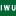Illinois Wesleyan University

#### A Categorical Semantics For Fuzzy Predicate Logic, Lawrence Stout

##### Scholarship

The object of this study is to look at categorical approaches to many valued logic, both propositional and predicate, to see how different logical properties result from different parts of the situation. In particular, the relationship between the categorical fabric I introduced at Linz in 2004 and the Fuzzy Logics studied by Hajek (2003) , Esteva et al. (2003) , and Hajek (1998) , comes from restricting the kind of structures used for truth values. We see how the structure of the various kinds of algebras shows up in the categorical logic, giving a variant on natural deduction for these ...

Categorical Approaches To Non-Commutative Fuzzy Logic, 2010Illinois Wesleyan University

#### Categorical Approaches To Non-Commutative Fuzzy Logic, Lawrence Stout

##### Scholarship

In this paper we consider what it means for a logic to be non-commutative, how to generate examples of structures with a non-commutative operation * which have enough nice properties to serve as the truth values for a logic. Inference in the propositional logic is gotten from the categorical properties (products, coproducts, monoidal and closed structures, adjoint functors) of the categories of truth values. We then show how to extend this view of propositional logic to a predicate logic using categories of propositions about a type A with functors giving change of type and adjoints giving quantifiers. In the case where ...

2010Illinois Wesleyan University

#### When Does A Category Built On A Lattice With A Monoidal Structure Have A Monoidal Structure?, Lawrence Stout

##### Scholarship

In a word, sometimes. And it gets harder if the structure on L is not commutative. In this paper we consider the question of what properties are needed on the lattice L equipped with an operation * for several different kinds of categories built using Sets and L to have monoidal and monoidal closed structures. This works best for the Goguen category Set(L) in which membership, but not equality, is made fuzzy and maps respect membership. Commutativity becomes critical if we make the equality fuzzy as well. This can be done several ways, so a progression of categories is considered ...

Hybridization And Postprocessing Techniques For Mixed Eigenfunctions, 2010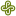University of Minnesota - Twin Cities

#### Hybridization And Postprocessing Techniques For Mixed Eigenfunctions, Bernardo Cockburn, Jay Gopalakrishnan, F. Li, Ngoc Cuong Nguyen, Jaume Peraire

##### Mathematics and Statistics Faculty Publications and Presentations

We introduce hybridization and postprocessing techniques for the Raviart–Thomas approximation of second-order elliptic eigenvalue problems. Hybridization reduces the Raviart–Thomas approximation to a condensed eigenproblem. The condensed eigenproblem is nonlinear, but smaller than the original mixed approximation. We derive multiple iterative algorithms for solving the condensed eigenproblem and examine their interrelationships and convergence rates. An element-by-element postprocessing technique to improve accuracy of computed eigenfunctions is also presented. We prove that a projection of the error in the eigenspace approximation by the mixed method (of any order) superconverges and that the postprocessed eigenfunction approximations converge faster for smooth eigenfunctions. Numerical ...

Coloring And Extremal Problems In Combinatorics, 2010Iowa State University

#### Coloring And Extremal Problems In Combinatorics, Jacob Manske

Coloring problems concern partitions of structures. The classic problem of partitioning the set of integers into a finite number of pieces so that no one piece has an arithmetic progression of a fixed length was solved in 1927. Van der Waerden's Theorem shows that it is impossible to do so. The theorem states that if the set of positive integers is partitioned into finitely many pieces, then at least one of the pieces contains arbitrarily long arithmetic progressions.

Extremal problems focus on finding the largest (or smallest) structures which exhibit a certain property. For instance, we may wish to ...

High School Teachers Use Of Dynamic Software To Generate Serendipitous Mathematical Relations, 2010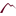University of Montana

#### High School Teachers Use Of Dynamic Software To Generate Serendipitous Mathematical Relations, Manuel Santos-Trigo, Hugo Espinosa-Pérez

##### The Mathematics Enthusiast

In this study, we document and analyse problem-solving approaches that high school teachers exhibited as a result of using dynamic software (Cabri-Geometry) to construct and examine geometric configurations. What type of questions do teachers pose and pursue while representing and exploring mathematical tasks or objects dynamically? To what extent their initial problem solving strategies are enhanced with the use of the tools? Results indicate that the use of the tool offered the participants the opportunity of constructing geometric configuration (formed by simple mathematical objects) that led them to identify and explore key mathematical relations.

“What Was Really Accomplished Today?” Mathematics Content Specialists Observe A Class For Prospective K–8 Teachers, 2010University of Montana

#### “What Was Really Accomplished Today?” Mathematics Content Specialists Observe A Class For Prospective K–8 Teachers, Andrew M. Tyminksi, Sarah Ledford, Dennis Hembree

##### The Mathematics Enthusiast

One of the important activities mathematics teacher educators engage in is the development of teachers at both the in-service and pre-service levels. Also of importance is the professional development of these professional developers. In the summer of 2004, a summer institute was held that allowed mathematics teacher educators watch the teaching of a mathematics content course for prospective K–8 teachers. This paper examines the manner in which a specific group of mathematics content specialists experienced this professional development.

Mathematical Reasoning In Service Courses: Why Students Need Mathematical Modeling Problems, 2010University of Montana

#### Mathematical Reasoning In Service Courses: Why Students Need Mathematical Modeling Problems, Kris H. Green, Allen Emerson

##### The Mathematics Enthusiast

In this paper we argue that conventional mathematics word problems are not aligned with the typical learning goals and expectations partner disciplines, especially business, have in requiring that their students take mathematics courses. Using the taxonomy of educational objectives presented by Anderson and Krathwohl (2001) we show how mathematical modeling problems can be used to promote the needed alignment and contrast two examples to illustrate the differences. We then demonstrate how the more conventional word problem can be rewritten as a modeling problem. Sample assessment materials and instructional activities are included to support teachers in making the transition to the ...

Leading Learning Within A Plc: Implementing New Mathematics Content, 2010University of Montana

#### Leading Learning Within A Plc: Implementing New Mathematics Content, Ann Heirdsfield, Janeen Lamb, Gayle Spry

##### The Mathematics Enthusiast

This paper does two things. Firstly, it examines the literature that coalesces around theoretical models of teacher professional development (PD) within a professional learning community (PLC). Secondly, these models are used to analyse support provided to two year 3 teachers, while implementing the draft Queensland mathematics syllabus. The findings from this study suggest that the development of this small PLC extended the teachers’ Zone of Enactment which in turn led to teacher action and reflection. This was demonstrated by the teachers leading their own learning as well as that of their students.

A Class Of Discontinuous Petrov–Galerkin Methods. Part I: The Transport Equation, 2010University of Texas at Austin

#### A Class Of Discontinuous Petrov–Galerkin Methods. Part I: The Transport Equation, Leszek Demkowicz, Jay Gopalakrishnan

##### Mathematics and Statistics Faculty Publications and Presentations

Considering a simple model transport problem, we present a new finite element method. While the new method fits in the class of discontinuous Galerkin (DG) methods, it differs from standard DG and streamline diffusion methods, in that it uses a space of discontinuous trial functions tailored for stability. The new method, unlike the older approaches, yields optimal estimates for the primal variable in both the element size h and polynomial degree p, and outperforms the standard upwind DG method.

Packing Rates Of Measures And A Conjecture For The Packing Density Of 2413, 2010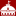Swarthmore College

#### Packing Rates Of Measures And A Conjecture For The Packing Density Of 2413, C. B. Presutti, Walter Stromquist

##### Mathematics & Statistics Faculty Works

We give a new lower bound of 0.10472422757673209041 for the packing density of 2413, justify it by a construction, and conjecture that this value is actually equal to the packing density. Along the way we define the packing rate of a permutation with respect to a measure, and show that maximizing the packing rate of a pattern over all measures gives the packing density of the pattern.

Edge Coloring Bibds And Constructing Moelrs , 2010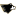Michigan Technological University

#### Edge Coloring Bibds And Constructing Moelrs , John S. Asplund

##### Dissertations, Master's Theses and Master's Reports - Open

Chapter 1 is used to introduce the basic tools and mechanics used within this thesis. Some historical uses and background are touched upon as well. The majority of the definitions are contained within this chapter as well.

In Chapter 2 we consider the question whether one can decompose λ copies of monochromatic Kv into copies of Kk such that each copy of the Kk contains at most one edge from each Kv. This is called a proper edge coloring (Hurd, Sarvate, ). The majority of the content in this section is a wide variety of examples ...

Numerical Investigation Of Aeroelastic Mode Distribution For Aircraft Wing Model In Subsonic Air Flow, 2010University of New Hampshire

#### Numerical Investigation Of Aeroelastic Mode Distribution For Aircraft Wing Model In Subsonic Air Flow, Marianna A. Shubov, Stephen B. Wineberg, Robert Holt

##### Mathematics & Statistics Scholarship

In this paper, the numerical results on two problems originated in aircraft wing modeling have been presented. The first problem is concerned with the approximation to the set of the aeroelastic modes, which are the eigenvalues of a certain boundary-value problem. The affirmative answer is given to the following question: can the leading asymptotical terms in the analytical formulas be used as reasonably accurate description of the aeroelastic modes? The positive answer means that these leading terms can be used by engineers for practical calculations. The second problem is concerned with the flutter phenomena in aircraft wings in a subsonic ...

2010University of Montana

#### Gender And Mathematics Education In Pakistan: A Situation Analysis, Anjum Halai

##### The Mathematics Enthusiast

This paper reports from a situation analysis of gender issues in mathematics education in Pakistan. It was undertaken at the initiation of a large scale project which aimed to understand how curriculum change in mathematics and science education may be implemented in ways that contribute to poverty alleviation and promote gender equity in disadvantaged rural settings. The paper posits that issues of access, achievement and quality of mathematics education are integrally linked with questions of equity, in this case gender equity. It identifies several questions and arenas for further research and makes recommendations for policy and practice in mathematics education.

2010University of Montana

#### Randomness: Developing An Understanding Of Mathematical Order, Steve Humble

##### The Mathematics Enthusiast

Is randomness merely the human inability to recognise a pattern that may in fact exist?

The three activities described show how order can be found in seemly random activities. The author has found that by using these activities on randomness, his students have developed a greater understanding of mathematical pattern and sequence. The teaching mathematical concepts in this way, engages and reinforces learning. It puts the ideas learnt into a setting and allows time for those ideas to be developed without any of the maths hang-ups which can sometimes occur in the classroom. By taking the maths beyond the classroom ...

Covariant Quantum Fields On Noncommutative Spacetimes, 2010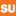Syracuse University

#### Covariant Quantum Fields On Noncommutative Spacetimes, A. P. Balachandran, A. Ibort, G. Marmo, M. Martone

##### Physics

A spinless covariant field $\phi$ on Minkowski spacetime $\M^{d+1}$ obeys the relation $U(a,\Lambda)\phi(x)U(a,\Lambda)^{-1}=\phi(\Lambda x+a)$ where $(a,\Lambda)$ is an element of the Poincar\'e group $\Pg$ and $U:(a,\Lambda)\to U(a,\Lambda)$ is its unitary representation on quantum vector states. It expresses the fact that Poincar\'e transformations are being unitary implemented. It has a classical analogy where field covariance shows that Poincar\'e transformations are canonically implemented. Covariance is self-reproducing: products of covariant fields are covariant. We recall these properties and use them to ...

Quantum Geons And Noncommutative Spacetimes, 2010Syracuse University

#### Quantum Geons And Noncommutative Spacetimes, A. P. Balachandran, A. Ibort, G. Marmo, M. Martone

##### Physics

Physical considerations strongly indicate that spacetime at Planck scales is noncommutative. A popular model for such a spacetime is the Moyal plane. The Poincare group algebra acts on it with a Drinfel'd-twisted coproduct. But the latter is not appropriate for more complicated spacetimes such as those containing the Friedman-Sorkin (topological) geons. They have rich diffeomorphism groups and in particular mapping class groups, so that the statistics groups for N identical geons is strikingly different from the permutation group SN. We generalise the Drinfel'd twist to (essentially) generic groups including to finite and discrete ones and use it to ...

2010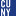CUNY Kingsborough Community College

#### Multiresolution Inverse Wavelet Reconstruction From A Fourier Partial Sum, Nataniel Greene

##### Publications and Research

The Gibbs phenomenon refers to the lack of uniform convergence which occurs in many orthogonal basis approximations to piecewise smooth functions. This lack of uniform convergence manifests itself in spurious oscillations near the points of discontinuity and a low order of convergence away from the discontinuities.In previous work [11,12] we described a numerical procedure for overcoming the Gibbs phenomenon called the Inverse Wavelet Reconstruction method (IWR). The method takes the Fourier coefficients of an oscillatory partial sum and uses them to construct the wavelet coefficients of a non-oscillatory wavelet series. However, we only described the method standard wavelet ...

The Maximum Rectilinear Crossing Number Of The Petersen Graph, 2010CUNY Kingsborough Community College

#### The Maximum Rectilinear Crossing Number Of The Petersen Graph, Elie Feder, Heiko Harborth, Steven Herzberg, Sheldon Klein

##### Publications and Research

We prove that the maximum rectilinear crossing number of the Petersen graph is 49. First, we illustrate a picture of the Petersen graph with 49 crossings to prove the lower bound. We then prove that this bound is sharp by carefully analyzing the ten Cs's which occur in the Petersen graph and their properties.